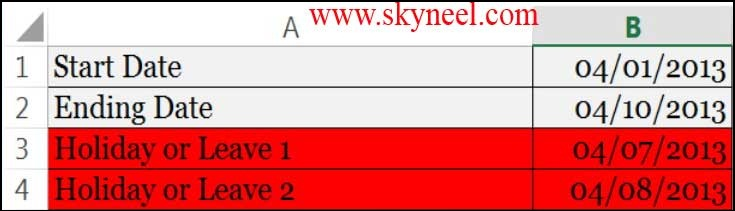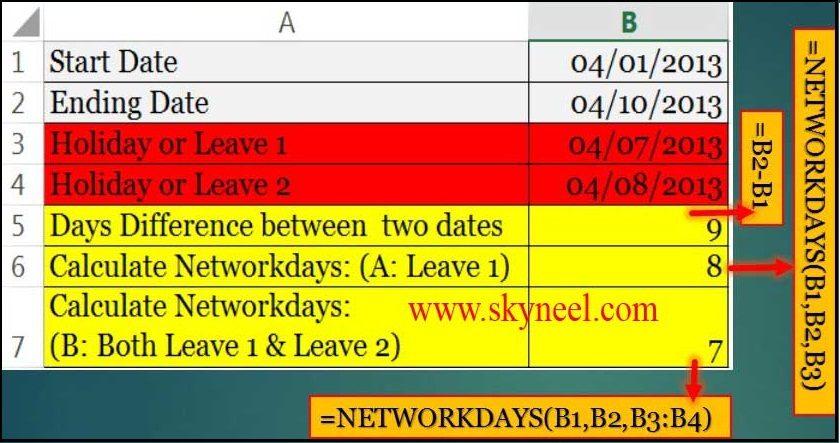# Count Working Days between Two Dates – MS Excel

NETWORKDAYS is a very powerful function in MS Excel’s which shown in date and time category. It is used to count working days between two dates.

Hi guys, we know very well about the importance of MS Excel. MS Excel is a electronic spreadsheet software. It is used for data analysis, modeling, solving complex calculations with the help of functions. There are lot of inbuilt functions in MS Excel. Today we discuss how to count working days between two dates in MS Excel. “NETWORKDAYS” is a very powerful formula in MS Excel’s date and time category which returns whole working days between given two dates, but there is one problem with it. It assumes 5 day workweek starting with Monday to Friday. Not all countries have workweek from Monday to Friday.

### Steps to Count Working Days between Two Dates

You must have to take few important steps to count working days between two dates in MS Excel. But before it you must have to know about the Networkdays function.

### Syntax NETWORKDAYS function:

NETWORKDAYS(start_date,end_date,holidays)
start_date is a date that represent the starting date.
end_date is a date that represent the ending date.
holidays: It is an optional part. In this part you can give any one date or range of more than one dates which represent the holidays and omitted from the functioning calendar, such as state and national holidays and variable holidays.

For example: If you are working in a company and you want to count working days between two dates in MS Excel. And also exclude all holidays or leaves from your total working days, in that type of condition you have to take following few simple steps to solve your problem

Step 1:Prepare the following data in the active sheet A1:B4Step 2: Now, type “Days difference between two dates” in cell A5. To calculate the result you have to type following formula in cell B5  =B2-B1. You will get the final result 9.

Step 3: Type “Calculate Networkdays: (A: Leave 1)” in cell A6 and type the following formula to get the result between two given dates in cell B6 =NETWORKDAYS(B1,B2,B3). When you press enter you get the final result 8 after deducting 1 holiday from total working days.

Step 4: Type “Calculate Networkdays: (B: Both Leave 1 & Leave 2)” in cell A7 and type following given formula to get the result between two given dates in cell B7 is: =NETWORKDAYS(B1,B2,B3:B4). After typing this formula, just you press enter key to get the final result 7 after deducting two holidays from total working days.